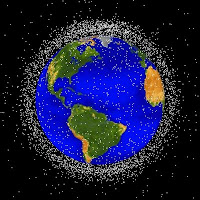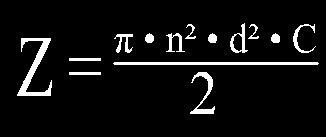# HEY! I’m Orbiting Here!UPDATED: Math corrected from yesterday’s typos, see note at the bottom under “comments.” Also the Colorado based Center for Space Standards & Innovation put out a great simulation of the crash, you can see the video here.

UPDATED UPDATE: Well, looks like I wasn’t the only one to goof on the collision math at first.

Kablammo! Like something out of a Bruce Willis movie, an American and a Russian satellite collided about 800 km above Siberia. The two blew apart into hundreds of pieces after smacking into each other at over 28,000 km per hour. It must’ve been one heck of a demolition derby up there.

Even though there are over 17,000 man-made objects over 10 cm in orbit, this is the first time two satellites have accidentally collided. Two years ago China intentionally shot one of theirs out of the sky. NORAD is constantally tracking all satellites in the skies, so they can predict when the big ones are going to hit. We’re interested in the odds of a random crash and it’s clear that the odds of two satellites accidentally hitting each other is very low. How low you may ask? Lets try to figure it out.

When we’re calculating the frequency of hits for a satellite we need to start out with some facts and how they’ll interact. We’ll start out by borrowing an equation from molecular physics calculating the odds of two molecules colliding.Here “Z” is the frequency of collisions for a single particle (satellites in this case). “n” represents the density of particles, “d” represents the size of the particles and “C” represents their average speed. The entire equation is divided by 2 to prevent double counting of collisions.

We can find the density of satellites by taking their total number divided by the volume of space they orbit in. Most satellites orbit in spheres between 500 and 1300 km above the surface of Earth. The equation V = 4/3 π r^3 gives us the volume of a sphere. Earth is about 6,300 km in diameter, making its radius (r) 3,150 km. First we find the volume of space the lowest orbiting satellites are in and subtract it from the highest orbiting satellites getting a total volume of space satellites orbit in.Over 165 billion cubic km is a lot of space. Density here is simply number of satellites per volume. 17,000 / 165,348,213,000 = 1.02813328 × 10^-7 satellites per km^3.

Finding their diameter is just a matter of estimating (we’ll assume every satellite is spherical here for simplicity’s sake). Satellites can run from small bits of space junk only 10 cm in diameter, to the International Space Station which is almost as big as a football field. For the sake of simplicity, ill round and say the average satellite size is 10 meters in diameter, or .01 km so we can keep all of our units straight.

Speeds for satellites vary based on their distance from the Earth. Again for the sake of simplicity, we’ll assume all satellites are orbiting at the same speed of 28,000 km/hr. That’s all we should need to find out how often satellites should be running into each other. We start out by plugging our numbers into the original equation:Giving us the result that an individual satellite will collide with another on average once every: 4.64682715 × 10^14 hours or roughly about once 53 billion years.

That’s the odds for one particular satellite, but all 17,000 would have roughly the same odds. Therefore : 4.64682715 × 10^14 hours / 17,000 = 27,334,277,400 hours or once every …So, not terribly common at all. This is only the roughest of calculations to figure this collisions conundrum. In fact collisions might be be more likely because these basic equations assume that all space junk is equally distributed, when in fact much if it is concentrated around the equator. The pull of gravity also factors in, as two bodies of junk will attract one another.

On the other hand, the equation I used is for the random Brownian motion of molecules, rather than the ordered orbits of satellites in the sky. Molecules are much freer to move in 3 dimensions, while satellites are mostly trapped at a single altitude. That way, higher satellites are much less likely to interact with lower ones.

But for a back of the envelope, off the top of my head, rule of thumb calculation, that’s pretty close without bringing in calculus and probability curves and the like. Let me know what you think. Am I close, or am I just making stuff up as I go?